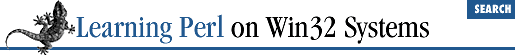home | O'Reilly's CD bookshelfs | FreeBSD | Linux | Cisco | Cisco Exam

### A.5 Chapter 6, Basic I/O

1. Here's one way to do it:

```print

reverse <>;```

You may be surprised at the brevity of this answer, but this answer will get the job done. Here's what is happening, from the inside out:

First, the ``` reverse``` operator is looking for a list for its arguments. Accordingly, the diamond operator (``` <>``` ) is being evaluated in a list context. Thus, all of the lines of the files named by command-line arguments (or standard input, if none are named) are read in, and then massaged into a list with one line per element.

Next, the ``` reverse``` operator reverses the list end-for-end.

Finally, the ``` print``` operator takes the resulting list, and displays it.

2. Here's one way to do it:

```@ARGV = reverse @ARGV;
print reverse <>;```

The first line just takes any filename arguments and reverses them. That way if the user called this script with command line arguments "camel gecko alpaca", ``` @ARGV``` would then contain "alpaca gecko camel" instead. The second line reads in all the lines in all the files in ``` @ARGV``` , flips them end on end, and prints them. If no arguments were passed to the program, then as before, <> works on STDIN instead.

3. Here's one way to do it:

```print "List of strings:\n";
chomp(@strings =

<STDIN>);

foreach (@strings) {
printf "%20s\n", \$_;
}```

The first line prompts for a list of strings.

The next line reads all of the strings into one array, and gets rid of the newlines at the end of each line.

The ``` foreach``` loop steps through this array, giving ``` \$_``` the value of each line.

The ``` printf``` operator gets two arguments: the first argument defines the format - ``` %20s\n``` means a 20-character right-justified column, followed by a newline.

4. Here's one way to do it:

```print "Field width: ";
chomp(\$width = <STDIN>);
print "List of strings:\n";
chomp(@strings = <STDIN>);
foreach (@strings) {

printf "%\${width}s\n", \$_;
}```

To the previous exercise's answer, we've added a prompt and response for the field width.

The other change is that the ``` printf``` format string now contains a variable reference. The value of ``` \$width``` is included into the string before ``` printf``` considers the format. Note that we cannot write this string as:

`printf "%\$widths\n", \$_; # WRONG`

because then Perl would be looking for a variable named ``` \$widths``` , not a variable named ``` \$width``` to which we attach an ``` s``` . Another way to write this is:

`printf "%\$width"."s\n", \$_; # RIGHT`

because the termination of the string also terminates the variable name, protecting the following character from being sucked up into the name.A.4 Chapter 5, HashesA.6 Chapter 7, Regular Expressions